# Math in Focus Grade 1 Cumulative Review Chapters 14 and 15 Answer Key

Go through the Math in Focus Grade 1 Workbook Answer Key Cumulative Review Chapters 14 and 15 to finish your assignments.

## Math in Focus Grade 1 Cumulative Review Chapters 14 and 15 Answer Key

Concepts and Skills

Use doubles facts or doubles plus one facts.

Question 1.
6 + 6 = ______
6+6 = 12.

Explanation:
The addition of 6+6 is 12.

Question 2.
8 + 7 = ______
8+7 = 7+7+1 = 15.

Explanation:
The double plus one fact for 8+7 is 7+7+1 which is 15.

Question 3.
12 + 5 = ______
12+5 = 17.

Explanation:
Here while adding mentally, we go  for a count of 5 numbers ahead. Which will be
12+1+1+1+1+1 = 12,13,14,15,16,17.

Question 4.
24 + 3 = _____
24+3 = 27.

Explanation:
Here while adding mentally, we go for a count of 3 numbers ahead. Which will be
24+1+1+1 = 24,25,26,27.

Question 5.
21 + 8 = ______
21+8 = 29.

Explanation:
Here while adding mentally, we go for a count of 8 numbers ahead. Which will be
21+1+1+1+1+1+1+1+1 = 21,22,23,24,25,26,27,28,29.

Question 6.
32 + 4 = _____
32+4 = 36.

Explanation:
Here while adding mentally, we go for a count of 4 numbers ahead. Which will be
32+1+1+1+1 = 32,33,34,35,36.

Question 7.
10 + 23 = ____
10 + 23 = 23.

Explanation:
Here while adding mentally, we will split into tens and ones, and then we will add them. Which will be
10 + 23 = 10+20+3
= 30+3
= 33.

Question 8.
18 + 10 = ____
18+10 = 28.

Explanation:
Here while adding mentally, we will split into tens and ones, and then we will add them. Which will be
18 + 10 = 10+10+8
= 20 + 8
= 28.

Subtract mentally.

Question 9.
11 – 5 = ___
11-5 = 6.

Explanation:
Here while subtracting mentally, we will go for a backward count of 5 numbers. Which will be
11-1,1,1,1,1 = 11,10,9,8,7,6.

Question 10.
18 – 9 = ____
18-9 = 9.

Explanation:
Here while subtracting mentally, we will go for a backward count of 9 numbers. Which will be
18-1-1-1-1-1-1-1-1-1 which is 18,17,16,15,14,13,12,11,10,9.

Question 11.
23 – 2 = ____
23-2 = 21.

Explanation:
Here while subtracting mentally, we will go for a backward count of 2 numbers. Which will be
23-1,1 which is 23,22,21.

Question 12.
27 – 2 = ___
27-2 = 25.

Explanation:
Here while subtracting mentally, we will go for a backward count of 2 numbers. Which will be
27-1,1 which is 27,26,25.

Subtract mentally.

Question 13.
26 – 10 = ___
26 – 10 = 16.

Explanation:
The mental subtraction of 26-10 is
26 – 10 = (20+6) -10
= 20-10+6
= 10+6
= 16.

Question 14.
35 – 10 = ____
35 – 10 = 15.

Explanation:
The mental subtraction of 35-10 is
35-10 = (30-5) – 10
= 30 – 10 – 5
= 20 – 5
= 15.

Question 15.
30 – 20 = ____
30 – 20 = 10.

Explanation:
The mental subtraction of 30 – 20 is 10.

Question 16.
27 – 20 = ____
27 – 20 = 7.

Explanation:
The mental subtraction of 27 – 20 is
27 – 20 = (20 + 7) – 20
= 20 – 20 + 7
= 7.

Fill in the blanks.

Question 17.
There are ____ months in one year.
There are 12 months in one year.

Question 18.
____ is the sixth month of the year.
June is the sixth month of the year.

Question 19.
_____ is the month that comes before December.
November is the month that comes before December.

Question 20.
____ months have 31 days.
7 months have 31 days.

Question 21.
The four seasons are spring, ____, ____, and ____.
The four seasons are spring, summer, fall, and winter.

Match.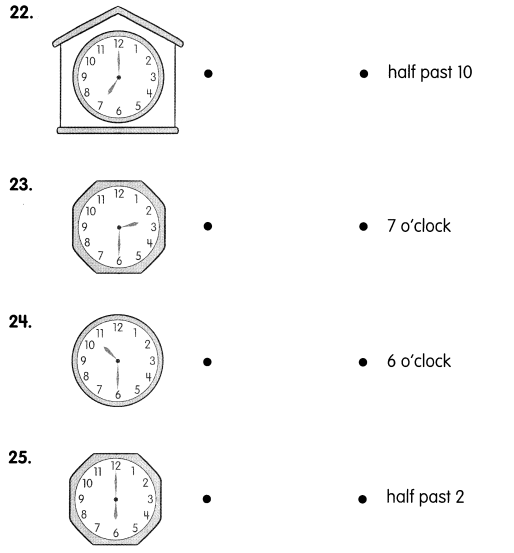Explanation: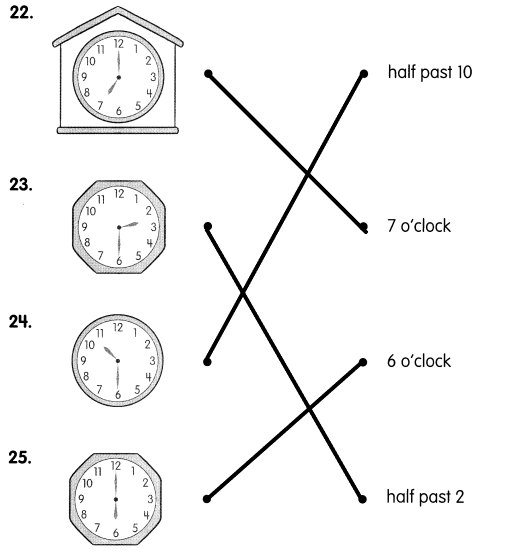Question 26.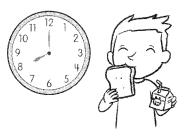Ethan eats his breakfast at ___.
Ethan eats his breakfast at 8:00.

Question 27.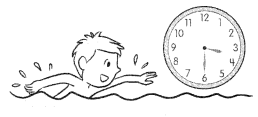Question 28.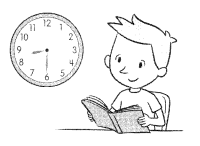Ethan reads his book at _____________

Ethan reads his book at 8:30.

Which clock shows the correct time?
Put a ✓ in the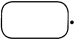Question 29.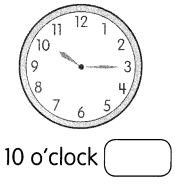No.

Explanation:
the clock shows 10:15.

Question 30.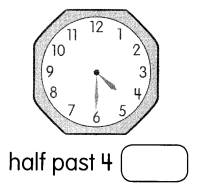Yes.

Explanation:
Yes, the clock shows half past 4.

Problem Solving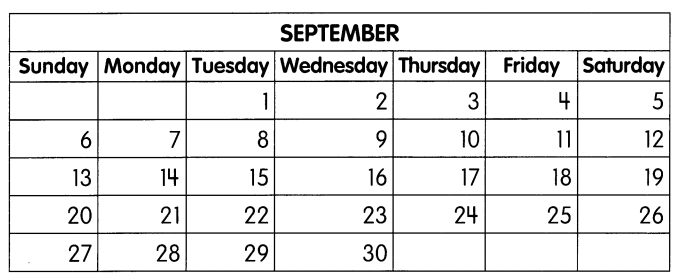Fill in the blanks.

Question 31.
The third day of the month falls on a _____
Thursday.

Explanation:
The third day of the month falls on a Thursday.

Question 32.
There are _____ days in this month.
30 days.

Explanation:
There are 30 days in this month.

Question 33.
The date of the third Thursday of the month is _____
17th.

Explanation:
The date of the third Thursday of the month is 17.

Question 34.
The day of the week after September 15 is _______
Wednesday.

Explanation:
The day of the week after September 15 is Wednesday.

Question 35.
The first day of September is on a ________.
So, the last day of August was on a ______________
Tuesday, Monday.

Explanation:
The first day of September is on a Tuesday.
So, the last day of August was on a Monday.

Use the calendar on pg 133.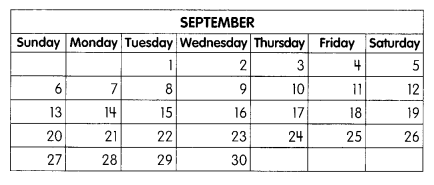Question 36.
___, ____, ____, _____

Question 37.
The date for each Friday is ________ more than the date for the Friday before.
7.

Explanation:
The date for each Friday is 7 more than the date for the Friday before.

Question 38.
This is because there are ____ days in a week.
7.

Explanation:
This is because there are 7 days in a week.

Problem Solving

Solve.